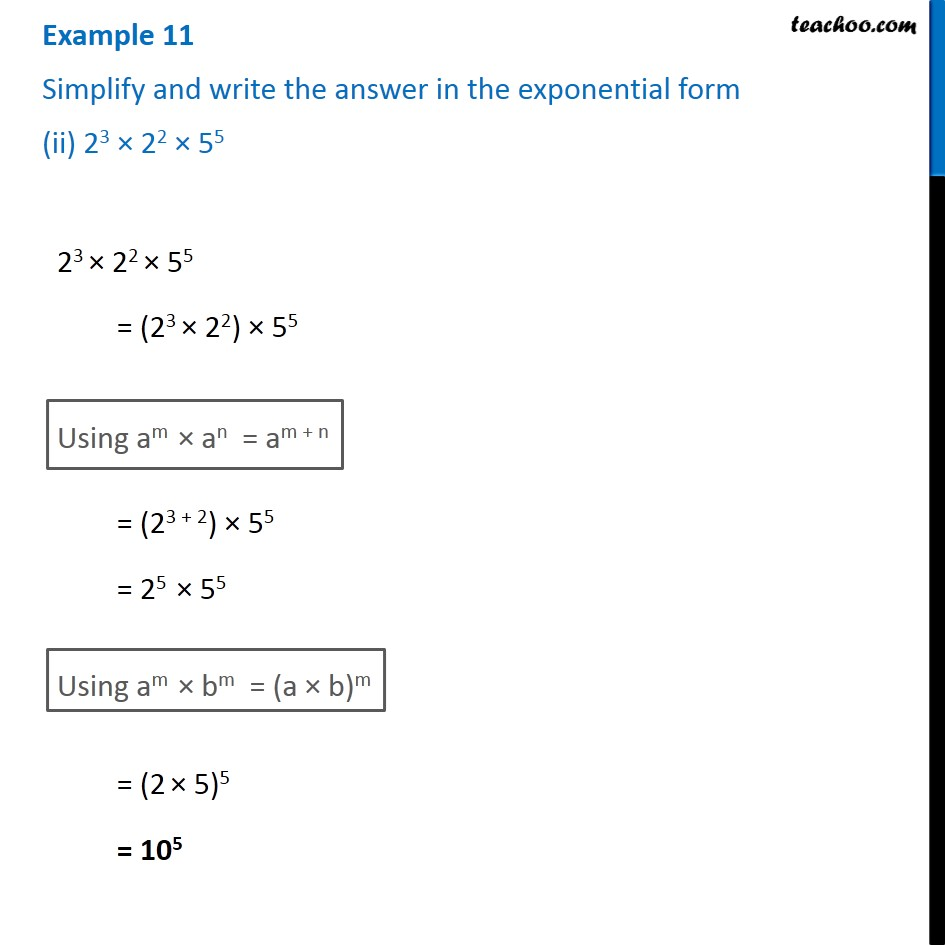1. Chapter 13 Class 7 Exponents and Powers
2. Serial order wise
3. Examples

Transcript

Example 11 Simplify and write the answer in the exponential form (ii) 23 × 22 × 55 23 × 22 × 55 = (23 × 22) × 55 Using am × an = am + n = (23 + 2) × 55 = 25 × 55 Using am × bm = (a × b)m = (2 × 5)5 = 105